# Tag: Kontsevich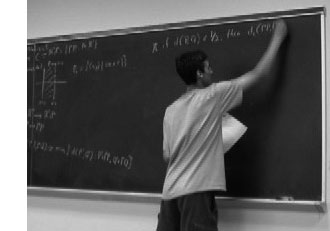Charles Siegel of Rigorous Trivialities ran a great series on big theorems.

The series started january 10th 2008 with a post on Bezout’s theorem, followed by posts on Chow’s lemma, Serre duality, Riemann-Roch, Bertini, Nakayama’s lemma, Groebner bases, Hurwitz to end just before christmas with a post on Kontsevich’s formula.

Also at other blogs, 2008 was the year of series of long posts containing substantial pure mathematics.

Out of many, just two examples : Chris Schommer-Pries ran a three part series on TQFTs via planar algebras starting here, at the secret blogging seminar.
And, Peter Woit of Not Even Wrong has an ungoing series of posts called Notes on BRST, starting here. At the moment he is at episode nine.

It suffices to have a quick look at the length of any of these posts, to see that a great deal of work was put into these series (and numerous similar ones, elsewhere). Is this amount of time well spend? Or, should we focus on shorter, easier digestible math-posts?

What got me thinking was this merciless comment Charles got after a great series of posts leading up to Kontsevich’s formula :

“Perhaps you should make a New Years commitment to not be so obscurantist, like John Armstrong, and instead promote the public understanding of math!”

Well, if this doesn’t put you off blogging for a while, what will?

So, are we really writing the wrong sort of posts? Do math-blog readers only want short, flashy, easy reading posts these days? Or, is anyone out there taking notice of the hard work it takes to write such a technical post, let alone a series of them?

At first I was rather pessimistic about the probable answer to all these questions, but, fortunately we have Google Analytics to quantify things a bit.

Clearly I can only rely on the statistics for my own site, so I’ll treat the case of a recent post here : Mumford’s treasure map which tried to explain the notion of a generic point and how one might depict an affine scheme.

Here’s some of the Google Analytics data :The yellow function gives the number of pageviews for that post, the value ranges between 0 and 600 (the number to the right of the picture). In total this post was viewed 2470 times, up till now.

The blue function tells the average time a visitor spend reading that post, the numbers range between 0 and 8 minutes (the times to the left of the picture). On average the time-on-page was 2.24 minutes, so in all people spend well over 92 hours reading this one post! This seems like a good return for the time it took me to write it…

Some other things can be learned from this data. Whereas the number of page-views has two peaks early on (one the day it was posted, the second one when Peter Woit linked to it) and is now steadily decreasing, the time-on-page for the later visitors is substantially longer than the early readers.

Some of this may be explained (see comment below) by returning visits. Here is a more detailed picture (orange = new visits, green=returning visits, blue=’total’ whatever this means).All in all good news : there is indeed a market for longer technical math-posts and people (eventually) take time to read the post in detail.Of course, excellent math-blogs exist in every language imaginable, but my linguistic limitations restrict me to the ones written in English, French, German and … Dutch. Here a few links to Dutch (or rather, Flemish) math-blogs, in order of proximity :
Stijn Symens blog, Rudy Penne’s wiskunde is sexy (math is sexy), Koen Vervloesem’s QED.

My favorite one is wiskundemeisjes (‘math-chicks’ or ‘math-girls’), written by Ionica Smeets and Jeanine Daems, two reasearchers at Leiden University. Every month they have a post called “the favorite (living) mathematician of …” in which they ask someone to nominate and introduce his/her favorite colleague mathematician. Here some examples : Roger Penrose chooses Michael Atiyah, Robbert Dijkgraaf chooses Maxim Kontsevich, Frans Oort chooses David Mumford, Gunther Cornelissen chooses Yuri I. Manin, Hendrik Lenstra chooses Bjorn Poonen, etc. the full list is here or here. This series deserves a wider audience. Perhaps Ionica and Jeanine might consider translating some of these posts?

I’m certain their English is far better than mine, so here’s a feeble attempt to translate the one post in their series they consider a complete failure (it isn’t even listed in the category). Two reasons for me to do so : it features Matilde Marcolli (one of my own favorite living mathematicians) and Matilde expresses here very clearly my own take on popular-math books/blogs.

The original post was written by Ionica and was called Weg met de ‘favoriete wiskundige van…’ :“This week I did spend much of my time at the Fifth European Mathematical Congress in Amsterdam. Several mathematicians suggested I should have a chat with Matilde Marcolli, one of the plenary speakers. It seemed like a nice idea to ask her about her favorite (still living) mathematician, for our series.

Marcolli explained why she couldn’t answer this question : she has favorite mathematical ideas, but it doesn’t interest her one bit who discovered or proved them. And, there are mathematicians she likes, but that’s because she finds them interesting as human beings, independent of their mathematical achievements.

In addition, she thinks it’s a mistake to focus science too much on the persons. Scientific ideas should play the main role, not the scientists themselves. To her it is important to remember that many results are the combined effort of several people, that science doesn’t evolve around personalities and that scientific ideas are accessible to anyone.

Marcolli also dislikes the current trend in popular science writing: “I am completely unable to read popular-scientific books. As soon as they start telling anecdotes and stories, I throw away the book. I don’t care about their lives, I care about the real stuff.”

She’d love to read a popular science-book containing only ideas. She regrets that most of these books restrict to story-telling, but fail to disseminate the scientific ideas.”

Ionica then goes on to defend her own approach to science-popularization :

“… Probably, people will not know much about Galois-theory by reading about his turbulent life. Still, I can imagine people to become interested in ‘the real stuff’ after reading his biography, and, in this manner they will read some mathematics they wouldn’t have known to exist otherwise. But, Marcolli got me thinking, for it is true that almost all popular science-books focus on anecdotes rather than science itself. Is this wrong? For instance, do you want to see more mathematics here? I’m curious to hear your opinion on this.”

Even though my own approach is somewhat different, Ionica and Jeanine you’re doing an excellent job: “houden zo!”

Last time we tried to generalize the Connes-Consani approach to commutative algebraic geometry over the field with one element $\mathbb{F}_1$ to the noncommutative world by considering covariant functors

$N~:~\mathbf{groups} \rightarrow \mathbf{sets}$

which over $\mathbb{C}$ resp. $\mathbb{Z}$ become visible by a complex (resp. integral) algebra having suitable universal properties.

However, we didn’t specify what we meant by a complex noncommutative variety (resp. an integral noncommutative scheme). In particular, we claimed that the $\mathbb{F}_1$-‘points’ associated to the functor

$D~:~\mathbf{groups} \rightarrow \mathbf{sets} \qquad G \mapsto G_2 \times G_3$ (here $G_n$ denotes all elements of order $n$ of $G$)

were precisely the modular dessins d’enfants of Grothendieck, but didn’t give details. We’ll try to do this now.

For algebras over a field we follow the definition, due to Kontsevich and Soibelman, of so called “noncommutative thin schemes”. Actually, the thinness-condition is implicit in both Soule’s-approach as that of Connes and Consani : we do not consider R-points in general, but only those of rings R which are finite and flat over our basering (or field).

So, what is a noncommutative thin scheme anyway? Well, its a covariant functor (commuting with finite projective limits)

$\mathbb{X}~:~\mathbf{Alg}^{fd}_k \rightarrow \mathbf{sets}$

from finite-dimensional (possibly noncommutative) $k$-algebras to sets. Now, the usual dual-space operator gives an anti-equivalence of categories

$\mathbf{Alg}^{fd}_k \leftrightarrow \mathbf{Coalg}^{fd}_k \qquad A=C^* \leftrightarrow C=A^*$

so a thin scheme can also be viewed as a contra-variant functor (commuting with finite direct limits)

$\mathbb{X}~:~\mathbf{Coalg}^{fd}_k \rightarrow \mathbf{Sets}$

In particular, we are interested to associated to any {tex]k $-algebra$A $its representation functor :$\mathbf{rep}(A)~:~\mathbf{Coalg}^{fd}_k \rightarrow \mathbf{Sets} \qquad C \mapsto Alg_k(A,C^*) $This may look strange at first sight, but$C^* $is a finite dimensional algebra and any$n $-dimensional representation of$A $is an algebra map$A \rightarrow M_n(k) $and we take$C $to be the dual coalgebra of this image. Kontsevich and Soibelman proved that every noncommutative thin scheme$\mathbb{X} $is representable by a$k $-coalgebra. That is, there exists a unique coalgebra$C_{\mathbb{X}} $(which they call the coalgebra of ‘distributions’ of$\mathbb{X} $) such that for every finite dimensional$k $-algebra$B $we have$\mathbb{X}(B) = Coalg_k(B^*,C_{\mathbb{X}}) $In the case of interest to us, that is for the functor$\mathbf{rep}(A) $the coalgebra of distributions is Kostant’s dual coalgebra$A^o $. This is the not the full linear dual of$A $but contains only those linear functionals on$A $which factor through a finite dimensional quotient. So? You’ve exchanged an algebra$A $for some coalgebra$A^o $, but where’s the geometry in all this? Well, let’s look at the commutative case. Suppose$A= \mathbb{C}[X] $is the coordinate ring of a smooth affine variety$X $, then its dual coalgebra looks like$\mathbb{C}[X]^o = \oplus_{x \in X} U(T_x(X)) $the direct sum of all universal (co)algebras of tangent spaces at points$x \in X $. But how do we get the variety out of this? Well, any coalgebra has a coradical (being the sun of all simple subcoalgebras) and in the case just mentioned we have$corad(\mathbb{C}[X]^o) = \oplus_{x \in X} \mathbb{C} e_x $so every point corresponds to a unique simple component of the coradical. In the general case, the coradical of the dual coalgebra$A^o $is the direct sum of all simple finite dimensional representations of$A $. That is, the direct summands of the coalgebra give us a noncommutative variety whose points are the simple representations, and the remainder of the coalgebra of distributions accounts for infinitesimal information on these points (as do the tangent spaces in the commutative case). In fact, it was a surprise to me that one can describe the dual coalgebra quite explicitly, and that$A_{\infty} $-structures make their appearance quite naturally. See this paper if you’re in for the details on this. That settles the problem of what we mean by the noncommutative variety associated to a complex algebra. But what about the integral case? In the above, we used extensively the theory of Kostant-duality which works only for algebras over fields… Well, not quite. In the case of$\mathbb{Z} $(or more general, of Dedekind domains) one can repeat Kostant’s proof word for word provided one takes as the definition of the dual$\mathbb{Z} $-coalgebra of an algebra (which is$\mathbb{Z} $-torsion free)$A^o = { f~:~A \rightarrow \mathbb{Z}~:~A/Ker(f)~\text{is finitely generated and torsion free}~} $(over general rings there may be also variants of this duality, as in Street’s book an Quantum groups). Probably lots of people have come up with this, but the only explicit reference I have is to the first paper I’ve ever written. So, also for algebras over$\mathbb{Z} $we can define a suitable noncommutative integral scheme (the coradical approach accounts only for the maximal ideals rather than all primes, but somehow this is implicit in all approaches as we consider only thin schemes). Fine! So, we can make sense of the noncommutative geometrical objects corresponding to the group-algebras$\mathbb{C} \Gamma $and$\mathbb{Z} \Gamma $where$\Gamma = PSL_2(\mathbb{Z}) $is the modular group (the algebras corresponding to the$G \mapsto G_2 \times G_3 $-functor). But, what might be the points of the noncommutative scheme corresponding to$\mathbb{F}_1 \Gamma $??? Well, let’s continue the path cut out before. “Points” should correspond to finite dimensional “simple representations”. Hence, what are the finite dimensional simple$\mathbb{F}_1 $-representations of$\Gamma $? (Or, for that matter, of any group$G $) Here we come back to Javier’s post on this : a finite dimensional$\mathbb{F}_1 $-vectorspace is a finite set. A$\Gamma $-representation on this set (of n-elements) is a group-morphism$\Gamma \rightarrow GL_n(\mathbb{F}_1) = S_n $hence it gives a permutation representation of$\Gamma $on this set. But then, if finite dimensional$\mathbb{F}_1 $-representations of$\Gamma $are the finite permutation representations, then the simple ones are the transitive permutation representations. That is, the points of the noncommutative scheme corresponding to$\mathbb{F}_1 \Gamma $are the conjugacy classes of subgroups$H \subset \Gamma $such that$\Gamma/H $is finite. But these are exactly the modular dessins d’enfants introduced by Grothendieck as I explained a while back elsewhere (see for example this post and others in the same series).Amidst all LHC-noise, Yuri I. Manin arXived today an interesting paper Cyclotomy and analytic geometry over$\mathbb{F}_1 $. The paper gives a nice survey of the existent literature and focusses on the crucial role of roots of unity in the algebraic geometry over the non-existent field with one element$\mathbb{F}_1 $(in French called ‘F-un’). I have tried to do a couple of posts on F-un some time ago but now realize, reading Manin’s paper, I may have given up way too soon… At several places in the paper, Manin hints at a possible noncommutative geometry over$\mathbb{F}_1 $: This is the appropriate place to stress that in a wider context of Toen-Vaqui ‘Au-dessous de Spec Z’, or eventually in noncommutative$\mathbb{F}_1 $-geometry, teh spectrum of$\mathbb{F}_1 $loses its privileged position as a final object of a geometric category. For example, in noncommutative geometry, or in an appropriate category of stacks, the quotient of this spectrum modulo the trivial action of a group must lie below this spectrum. Soule’s algebras$\mathcal{A}_X $are a very important element of the structure, in particular, because they form a bridge to Arakelov geometry. Soule uses concrete choices of them in order to produce ‘just right’ supply of morphisms, without a priori constraining these choices formally. In this work, we use these algebras and their version also to pave a way to the analytic (and possibly non-commutative) geometry over$\mathbb{F}_1 $. Back when I was writing the first batch of F-un posts, I briefly contemplated the possibility of a noncommutative geometry over$\mathbb{F}_1 $, but quickly forgot about it because I thought it would be forced to reduce to commutative geometry. Here is the quick argument : noncommutative geometry is really the study of coalgebras (see for example my paper or if you prefer more trustworthy sources the Kontsevich-Soibelman paper). Now, unless I made a mistake, I think all coalgebras over$\mathbb{F}_1 $must be co-commutative (even group-like), so reducing to commutative geometry. Surely, I’m missing something… The Monster is the largest of the 26 sporadic simple groups and has order 808 017 424 794 512 875 886 459 904 961 710 757 005 754 368 000 000 000 = 2^46 3^20 5^9 7^6 11^2 13^3 17 19 23 29 31 41 47 59 71. It is not so much the size of its order that makes it hard to do actual calculations in the monster, but rather the dimensions of its smallest non-trivial irreducible representations (196 883 for the smallest, 21 296 876 for the next one, and so on). In characteristic two there is an irreducible representation of one dimension less (196 882) which appears to be of great use to obtain information. For example, Robert Wilson used it to prove that The Monster is a Hurwitz group. This means that the Monster is generated by two elements g and h satisfying the relations$g^2 = h^3 = (gh)^7 = 1 $Geometrically, this implies that the Monster is the automorphism group of a Riemann surface of genus g satisfying the Hurwitz bound 84(g-1)=#Monster. That is, g=9619255057077534236743570297163223297687552000000001=42151199 * 293998543 * 776222682603828537142813968452830193 Or, in analogy with the Klein quartic which can be constructed from 24 heptagons in the tiling of the hyperbolic plane, there is a finite region of the hyperbolic plane, tiled with heptagons, from which we can construct this monster curve by gluing the boundary is a specific way so that we get a Riemann surface with exactly 9619255057077534236743570297163223297687552000000001 holes. This finite part of the hyperbolic tiling (consisting of #Monster/7 heptagons) we’ll call the empire of the monster and we’d love to describe it in more detail.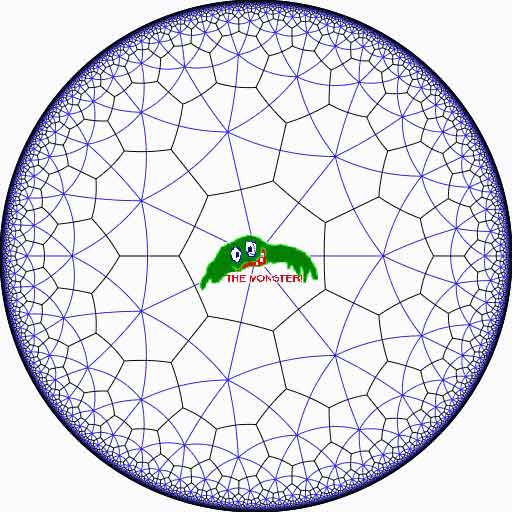Look at the half-edges of all the heptagons in the empire (the picture above learns that every edge in cut in two by a blue geodesic). They are exactly #Monster such half-edges and they form a dessin d’enfant for the monster-curve. If we label these half-edges by the elements of the Monster, then multiplication by g in the monster interchanges the two half-edges making up a heptagonal edge in the empire and multiplication by h in the monster takes a half-edge to the one encountered first by going counter-clockwise in the vertex of the heptagonal tiling. Because g and h generated the Monster, the dessin of the empire is just a concrete realization of the monster. Because g is of order two and h is of order three, the two permutations they determine on the dessin, gives a group epimorphism$C_2 \ast C_3 = PSL_2(\mathbb{Z}) \rightarrow \mathbb{M} $from the modular group$PSL_2(\mathbb{Z}) $onto the Monster-group. In noncommutative geometry, the group-algebra of the modular group$\mathbb{C} PSL_2 $can be interpreted as the coordinate ring of a noncommutative manifold (because it is formally smooth in the sense of Kontsevich-Rosenberg or Cuntz-Quillen) and the group-algebra of the Monster$\mathbb{C} \mathbb{M} $itself corresponds in this picture to a finite collection of ‘points’ on the manifold. Using this geometric viewpoint we can now ask the question What does the Monster see of the modular group? To make sense of this question, let us first consider the commutative equivalent : what does a point P see of a commutative variety X?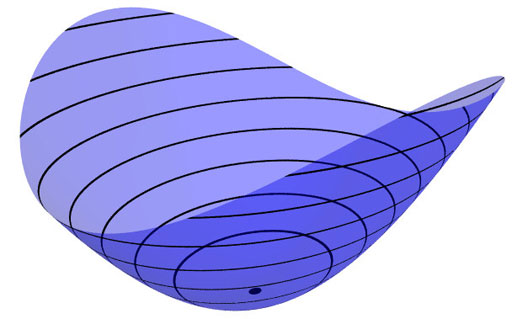Evaluation of polynomial functions in P gives us an algebra epimorphism$\mathbb{C}[X] \rightarrow \mathbb{C} $from the coordinate ring of the variety$\mathbb{C}[X] $onto$\mathbb{C} $and the kernel of this map is the maximal ideal$\mathfrak{m}_P $of$\mathbb{C}[X] $consisting of all functions vanishing in P. Equivalently, we can view the point$P= \mathbf{spec}~\mathbb{C}[X]/\mathfrak{m}_P $as the scheme corresponding to the quotient$\mathbb{C}[X]/\mathfrak{m}_P $. Call this the 0-th formal neighborhood of the point P. This sounds pretty useless, but let us now consider higher-order formal neighborhoods. Call the affine scheme$\mathbf{spec}~\mathbb{C}[X]/\mathfrak{m}_P^{n+1} $the n-th forml neighborhood of P, then the first neighborhood, that is with coordinate ring$\mathbb{C}[X]/\mathfrak{m}_P^2 $gives us tangent-information. Alternatively, it gives the best linear approximation of functions near P. The second neighborhood$\mathbb{C}[X]/\mathfrak{m}_P^3 $gives us the best quadratic approximation of function near P, etc. etc. These successive quotients by powers of the maximal ideal$\mathfrak{m}_P $form a system of algebra epimorphisms$\ldots \frac{\mathbb{C}[X]}{\mathfrak{m}_P^{n+1}} \rightarrow \frac{\mathbb{C}[X]}{\mathfrak{m}_P^{n}} \rightarrow \ldots \ldots \rightarrow \frac{\mathbb{C}[X]}{\mathfrak{m}_P^{2}} \rightarrow \frac{\mathbb{C}[X]}{\mathfrak{m}_P} = \mathbb{C} $and its inverse limit$\underset{\leftarrow}{lim}~\frac{\mathbb{C}[X]}{\mathfrak{m}_P^{n}} = \hat{\mathcal{O}}_{X,P} $is the completion of the local ring in P and contains all the infinitesimal information (to any order) of the variety X in a neighborhood of P. That is, this completion$\hat{\mathcal{O}}_{X,P} $contains all information that P can see of the variety X. In case P is a smooth point of X, then X is a manifold in a neighborhood of P and then this completion$\hat{\mathcal{O}}_{X,P} $is isomorphic to the algebra of formal power series$\mathbb{C}[[ x_1,x_2,\ldots,x_d ]] $where the$x_i $form a local system of coordinates for the manifold X near P. Right, after this lengthy recollection, back to our question what does the monster see of the modular group? Well, we have an algebra epimorphism$\pi~:~\mathbb{C} PSL_2(\mathbb{Z}) \rightarrow \mathbb{C} \mathbb{M} $and in analogy with the commutative case, all information the Monster can gain from the modular group is contained in the$\mathfrak{m} $-adic completion$\widehat{\mathbb{C} PSL_2(\mathbb{Z})}_{\mathfrak{m}} = \underset{\leftarrow}{lim}~\frac{\mathbb{C} PSL_2(\mathbb{Z})}{\mathfrak{m}^n} $where$\mathfrak{m} $is the kernel of the epimorphism$\pi $sending the two free generators of the modular group$PSL_2(\mathbb{Z}) = C_2 \ast C_3 $to the permutations g and h determined by the dessin of the pentagonal tiling of the Monster’s empire. As it is a hopeless task to determine the Monster-empire explicitly, it seems even more hopeless to determine the kernel$\mathfrak{m} $let alone the completed algebra… But, (surprise) we can compute$\widehat{\mathbb{C} PSL_2(\mathbb{Z})}_{\mathfrak{m}} $as explicitly as in the commutative case we have$\hat{\mathcal{O}}_{X,P} \simeq \mathbb{C}[[ x_1,x_2,\ldots,x_d ]] $for a point P on a manifold X. Here the details : the quotient$\mathfrak{m}/\mathfrak{m}^2 $has a natural structure of$\mathbb{C} \mathbb{M} $-bimodule. The group-algebra of the monster is a semi-simple algebra, that is, a direct sum of full matrix-algebras of sizes corresponding to the dimensions of the irreducible monster-representations. That is,$\mathbb{C} \mathbb{M} \simeq \mathbb{C} \oplus M_{196883}(\mathbb{C}) \oplus M_{21296876}(\mathbb{C}) \oplus \ldots \ldots \oplus M_{258823477531055064045234375}(\mathbb{C}) $with exactly 194 components (the number of irreducible Monster-representations). For any$\mathbb{C} \mathbb{M} $-bimodule$M $one can form the tensor-algebra$T_{\mathbb{C} \mathbb{M}}(M) = \mathbb{C} \mathbb{M} \oplus M \oplus (M \otimes_{\mathbb{C} \mathbb{M}} M) \oplus (M \otimes_{\mathbb{C} \mathbb{M}} M \otimes_{\mathbb{C} \mathbb{M}} M) \oplus \ldots \ldots $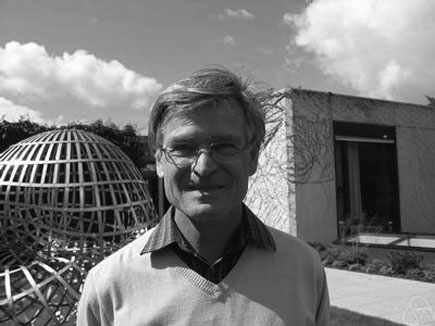and applying the formal neighborhood theorem for formally smooth algebras (such as$\mathbb{C} PSL_2(\mathbb{Z}) $) due to Joachim Cuntz (left) and Daniel Quillen (right) we have an isomorphism of algebras$\widehat{\mathbb{C} PSL_2(\mathbb{Z})}_{\mathfrak{m}} \simeq \widehat{T_{\mathbb{C} \mathbb{M}}(\mathfrak{m}/\mathfrak{m}^2)} $where the right-hand side is the completion of the tensor-algebra (at the unique graded maximal ideal) of the$\mathbb{C} \mathbb{M} $-bimodule$\mathfrak{m}/\mathfrak{m}^2 $, so we’d better describe this bimodule explicitly. Okay, so what’s a bimodule over a semisimple algebra of the form$S=M_{n_1}(\mathbb{C}) \oplus \ldots \oplus M_{n_k}(\mathbb{C}) $? Well, a simple S-bimodule must be either (1) a factor$M_{n_i}(\mathbb{C}) $with all other factors acting trivially or (2) the full space of rectangular matrices$M_{n_i \times n_j}(\mathbb{C}) $with the factor$M_{n_i}(\mathbb{C}) $acting on the left,$M_{n_j}(\mathbb{C}) $acting on the right and all other factors acting trivially. That is, any S-bimodule can be represented by a quiver (that is a directed graph) on k vertices (the number of matrix components) with a loop in vertex i corresponding to each simple factor of type (1) and a directed arrow from i to j corresponding to every simple factor of type (2). That is, for the Monster, the bimodule$\mathfrak{m}/\mathfrak{m}^2 $is represented by a quiver on 194 vertices and now we only have to determine how many loops and arrows there are at or between vertices. Using Morita equivalences and standard representation theory of quivers it isn’t exactly rocket science to determine that the number of arrows between the vertices corresponding to the irreducible Monster-representations$S_i $and$S_j $is equal to$dim_{\mathbb{C}}~Ext^1_{\mathbb{C} PSL_2(\mathbb{Z})}(S_i,S_j)-\delta_{ij} $Now, I’ve been wasting a lot of time already here explaining what representations of the modular group have to do with quivers (see for example here or some other posts in the same series) and for quiver-representations we all know how to compute Ext-dimensions in terms of the Euler-form applied to the dimension vectors. Right, so for every Monster-irreducible$S_i $we have to determine the corresponding dimension-vector$~(a_1,a_2;b_1,b_2,b_3) $for the quiver$\xymatrix{ & & & &
\vtx{b_1} \\ \vtx{a_1} \ar[rrrru]^(.3){B_{11}} \ar[rrrrd]^(.3){B_{21}}
\ar[rrrrddd]_(.2){B_{31}} & & & & \\ & & & & \vtx{b_2} \\ \vtx{a_2}
\ar[rrrruuu]_(.7){B_{12}} \ar[rrrru]_(.7){B_{22}}
\ar[rrrrd]_(.7){B_{23}} & & & & \\ & & & & \vtx{b_3}} $Now the dimensions$a_i $are the dimensions of the +/-1 eigenspaces for the order 2 element g in the representation and the$b_i $are the dimensions of the eigenspaces for the order 3 element h. So, we have to determine to which conjugacy classes g and h belong, and from Wilson’s paper mentioned above these are classes 2B and 3B in standard Atlas notation. So, for each of the 194 irreducible Monster-representations we look up the character values at 2B and 3B (see below for the first batch of those) and these together with the dimensions determine the dimension vector$~(a_1,a_2;b_1,b_2,b_3) $.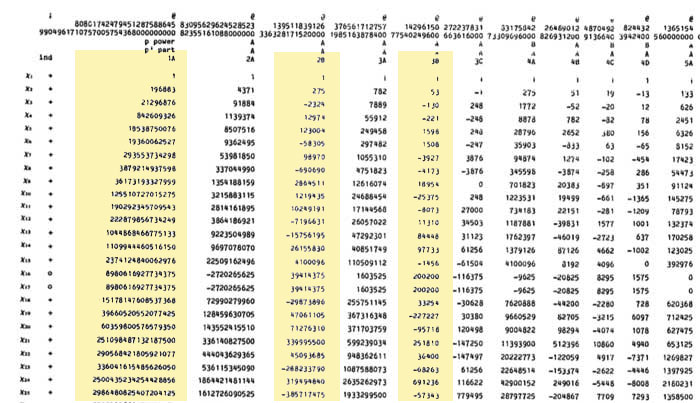For example take the 196883-dimensional irreducible. Its 2B-character is 275 and the 3B-character is 53. So we are looking for a dimension vector such that$a_1+a_2=196883, a_1-275=a_2 $and$b_1+b_2+b_3=196883, b_1-53=b_2=b_3 $giving us for that representation the dimension vector of the quiver above$~(98579,98304,65663,65610,65610) $. Okay, so for each of the 194 irreducibles$S_i $we have determined a dimension vector$~(a_1(i),a_2(i);b_1(i),b_2(i),b_3(i)) $, then standard quiver-representation theory asserts that the number of loops in the vertex corresponding to$S_i $is equal to$dim(S_i)^2 + 1 – a_1(i)^2-a_2(i)^2-b_1(i)^2-b_2(i)^2-b_3(i)^2 $and that the number of arrows from vertex$S_i $to vertex$S_j $is equal to$dim(S_i)dim(S_j) – a_1(i)a_1(j)-a_2(i)a_2(j)-b_1(i)b_1(j)-b_2(i)b_2(j)-b_3(i)b_3(j) $This data then determines completely the$\mathbb{C} \mathbb{M} $-bimodule$\mathfrak{m}/\mathfrak{m}^2 $and hence the structure of the completion$\widehat{\mathbb{C} PSL_2}_{\mathfrak{m}} $containing all information the Monster can gain from the modular group. But then, one doesn’t have to go for the full regular representation of the Monster. Any faithful permutation representation will do, so we might as well go for the one of minimal dimension. That one is known to correspond to the largest maximal subgroup of the Monster which is known to be a two-fold extension$2.\mathbb{B} $of the Baby-Monster. The corresponding permutation representation is of dimension 97239461142009186000 and decomposes into Monster-irreducibles$S_1 \oplus S_2 \oplus S_4 \oplus S_5 \oplus S_9 \oplus S_{14} \oplus S_{21} \oplus S_{34} \oplus S_{35} \$

(in standard Atlas-ordering) and hence repeating the arguments above we get a quiver on just 9 vertices! The actual numbers of loops and arrows (I forgot to mention this, but the quivers obtained are actually symmetric) obtained were found after laborious computations mentioned in this post and the details I’ll make avalable here.

Anyone who can spot a relation between the numbers obtained and any other part of mathematics will obtain quantities of genuine (ie. non-Inbev) Belgian beer…